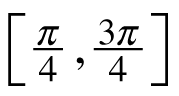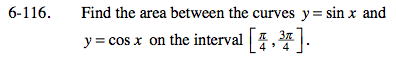### Home > CALC > Chapter 6 > Lesson 6.3.4 > Problem6-116

6-116.

Find the area between the curves y = sin x and y = cos x on the interval. Homework Help ✎You know the bounds. But do you know what happens between the bounds? Which function is on top and which is on the bottom?

Area between curves =

$\int_{A}^{B}((\text{top function})-(\text{bottom function}))dx$

A = left-most bound
B = right-most bound